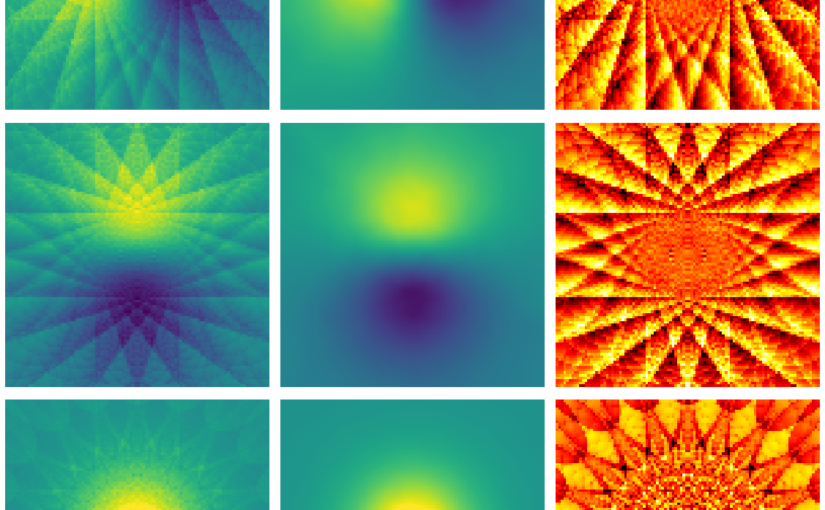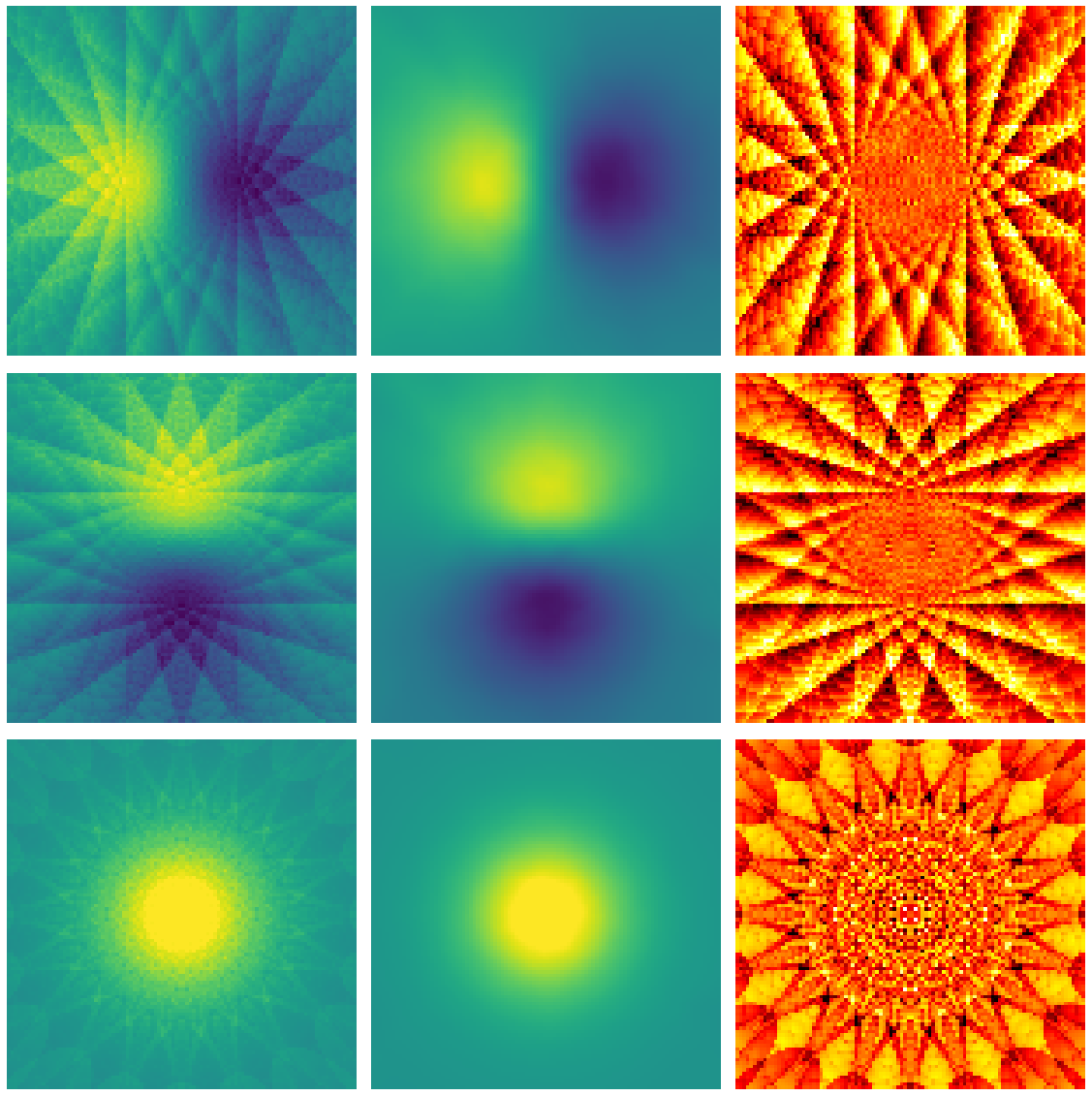# Presentation by D. Bronte Ciriza at OSA-OMA-2021Optical forces calculated on a sphere with the geometrical optics (left column) and the machine learning (center column) approaches. The difference between both approaches is shown in the column on the right, illustrating the removal of artefacts with the machine learning method.

Machine learning to enhance the calculation of optical forces in the geometrical optics approximation
David Bronte Ciriza, Alessandro Magazzù, Agnese Callegari, Maria A. Iatì, Giovanni Volpe, Onofrio M. Maragò
Submitted to OSA-OMA-2021, AF2D.2 Contribution
Date: 16 April
Time: 17 CEST

Short Abstract: We show how machine learning can improve the speed and accuracy of the optical force calculations in the geometrical optics approximation.

Extended Abstract:

Light can exert forces by exchanging momentum with particles. Since the pioneering work by Ashkin in the 1970’s, optical forces have played a fundamental role in fields like biology, nanotechnology, or atomic physics. Optical tweezers, which are instruments that, by tightly focusing a laser beam, are capable of confining particles in three dimensions, have become a common tool for manipulation of micro- and nano- particles, as well as a force and torque transducer with sensing capabilities at the femtonewton level. Optical tweezers have also been successfully employed to explore novel phenomena, including protein folding and molecular motors, or the optical forces and Brownian motion of 1D and 2D materials.

Numerical simulations play a fundamental role in the planning of experiments and in the interpretation of the results. In some basic cases for optical tweezers, the optical trap can be approximated by a harmonic potential. However, there are many situations where this approximation is insufficient, for example in the case of a particle escaping an optical trap, or for particles that are moving on an optical landscape but are not trapped. In these cases, a more complex treatment of the light-matter interaction is required for a more accurate calculation of the forces. This calculation is computationally expensive and prohibitively slow for numerical simulations when the forces need to be calculated many times in a sequential way. Recently, machine learning has been demonstrated to be a promising approach to improve the speed of these calculations and therefore, to expand the applicability of numerical simulations for experimental design and analysis.

In this work, we explore the geometrical optics regime, valid when the particles are significantly bigger than the wavelength of the incident light. This is typically the case in experiments with micrometer-size particles. The optical field is described by a collection of N light rays and the momentum exchange between the rays and the particle is calculated employing the tools of geometrical optics. The limitation of considering a discrete N number of light rays introduces artifacts in the force calculation. We show that machine learning can be used to improve not only the speed but also the accuracy of the force calculation. This is first demonstrated by training a neural network for the case of a spherical particle with 3 degrees of freedom accounting for the position of the particle. We show how the neural network improves the prediction of the force with respect to the initial training data that has been generated through the geometrical optics approach.
Starting from these results for 3 degrees of freedom, the work has been expanded to 9 degrees of freedom by including all the relevant parameters for the optical forces calculation considering also different refractive indexes, shapes, sizes, positions and orientations of the particle besides different numerical apertures of the objective that focuses the light.

This work proves machine learning as a compact, accurate, and fast approach for optical forces calculation and presents a tool that can be used to study systems that, due to computation limitations, were out of the scope of the traditional ray optics approach.

This site uses Akismet to reduce spam. Learn how your comment data is processed.